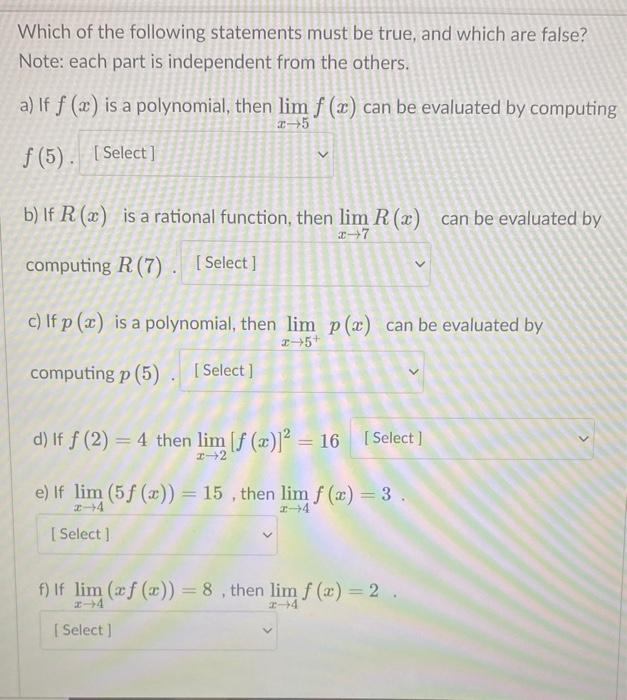# Question Solved1 AnswerWhich of the following statements must be true, and which are false? Note: each part is independent from the others. a) If f (2) is a polynomial, then lim f (2) can be evaluated by computing 05 f(5). [Select] b) If R(x) is a rational function, then lim R (2) can be evaluated by 2-7 computing R(7). [Select ] c) If p(x) is a polynomial, then lim p(x) can be evaluated by 3+5+ computing p (5) [Select] > d) If f (2) = 4 then lim (f (x)] = 16 [ Select) e) If lim (5 f (x)) = 15, then lim f (x) = 3. 24 [ Select ] f) If lim (af (x)) = 8 , then lim f (x) = 2 . 4 SelectTranscribed Image Text: Which of the following statements must be true, and which are false? Note: each part is independent from the others. a) If f (2) is a polynomial, then lim f (2) can be evaluated by computing 05 f(5). [Select] b) If R(x) is a rational function, then lim R (2) can be evaluated by 2-7 computing R(7). [Select ] c) If p(x) is a polynomial, then lim p(x) can be evaluated by 3+5+ computing p (5) [Select] > d) If f (2) = 4 then lim (f (x)] = 16 [ Select) e) If lim (5 f (x)) = 15, then lim f (x) = 3. 24 [ Select ] f) If lim (af (x)) = 8 , then lim f (x) = 2 . 4 Select
More
Transcribed Image Text: Which of the following statements must be true, and which are false? Note: each part is independent from the others. a) If f (2) is a polynomial, then lim f (2) can be evaluated by computing 05 f(5). [Select] b) If R(x) is a rational function, then lim R (2) can be evaluated by 2-7 computing R(7). [Select ] c) If p(x) is a polynomial, then lim p(x) can be evaluated by 3+5+ computing p (5) [Select] > d) If f (2) = 4 then lim (f (x)] = 16 [ Select) e) If lim (5 f (x)) = 15, then lim f (x) = 3. 24 [ Select ] f) If lim (af (x)) = 8 , then lim f (x) = 2 . 4 Select